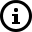# How is the disk utilization calculated for a Linux server monitor?

Disk utilization (both total disk utilization and partition disk utilization) is calculated by executing the following command:df -l -T

Consider the following sample output:disk_used_percent = (used_disk/total_disk) * 100

where,
used_disk = sum of the used spaces of all disk partitions
total_disk = sum of the used and available spaces of all disk partitions (Used + Available)

Note: The resulting value will be rounded off to two floating point values.